# How to Read a Capacitor?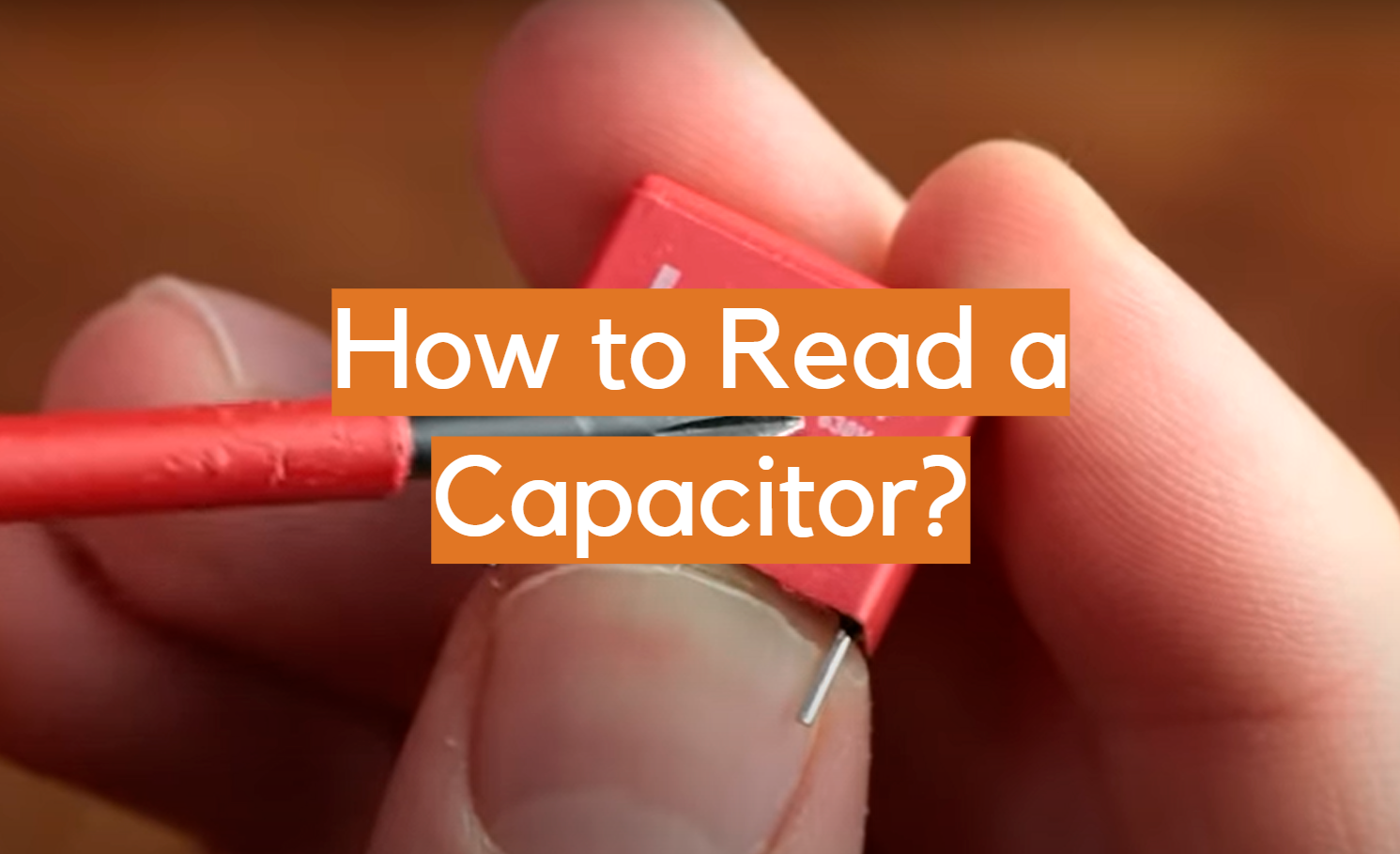A capacitor is an electronic component that stores energy in an electric field. It is used in many different applications, from computers and televisions to cars and trucks. If you have ever had to replace a capacitor, you know that they can be expensive. That’s why it’s important to be able to read one so you can determine whether it needs to be replaced or not. In this article, we will demystify the process of reading a capacitor so you can make an informed decision the next time you need to replace one.

## What is a Capacitor?

A capacitor is an electronic device that stores and releases electrical energy. Capacitors are found in a wide range of electronic devices, from computers to cell phones to cars. When charged, capacitors store energy in an electric field between two conductive plates. When discharged, they release this stored energy into the circuit. This can be used to power electronic devices or to provide a current boost when needed.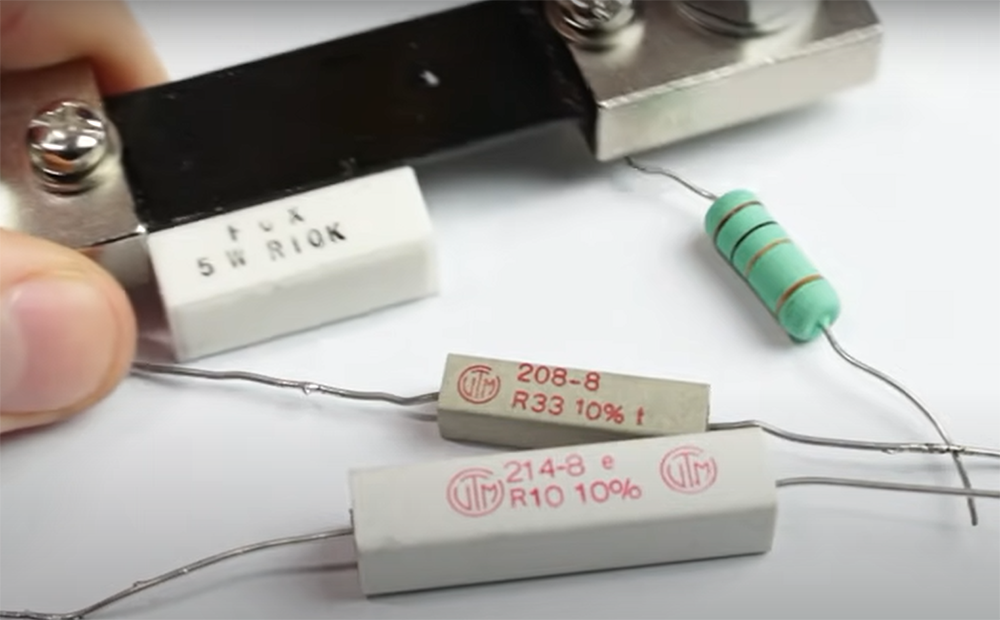Capacitors come in a variety of sizes and shapes, but all have two terminals that allow them to be connected to a circuit. The size of the capacitor (measured in farads) determines how much charge it can store. Capacitors are available in a wide range of sizes, from tiny disk capacitors that can fit in your pocket to large canister capacitors that are used in industrial applications.

There are two main types of capacitors: electrolytic and non-electrolytic. The most common type is the electrolytic capacitor, which consists of two metal plates separated by an electrolyte. These capacitors are polarized, meaning that they can only be connected one way in a circuit. If you connect them backwards, they will be destroyed. Another type of capacitor is the ceramic capacitor, which consists of two pieces of ceramic material separated by a thin layer of metal. Ceramic capacitors are not polarized and can be connected either way in a circuit.

Capacitors are often used to filter out unwanted electrical noise from a signal. They can also be used to store energy for short periods of time, such as in a camera flash or a car’s ignition system. When choosing a capacitor, it is important to consider the size, voltage rating, and capacitance (measured in farads). 

## Understand the Units of Measurement

Now that we’ve answered the question “what is a capacitor?”, let’s move on to how to read one. When reading a capacitor, you will need to know three things: the value, the tolerance, and the voltage rating.

Capacitors are usually labeled with their capacitance, which is measured in farads. The farad is a unit of measurement that represents the amount of charge a capacitor can store. One farad is equal to one coulomb (the SI unit of electric charge) per volt (the SI unit of electric potential). Farads are named after Michael Faraday, an English scientist who discovered electrolysis in 1834.

The capacitance of a capacitor is usually printed on the side of the component, along with the voltage rating and other information. For example, a capacitor might be labeled “100V” and “0.47uF”. This means that the capacitor has a capacitance of 0.47 microfarads (uF) and a voltage rating of 100 volts.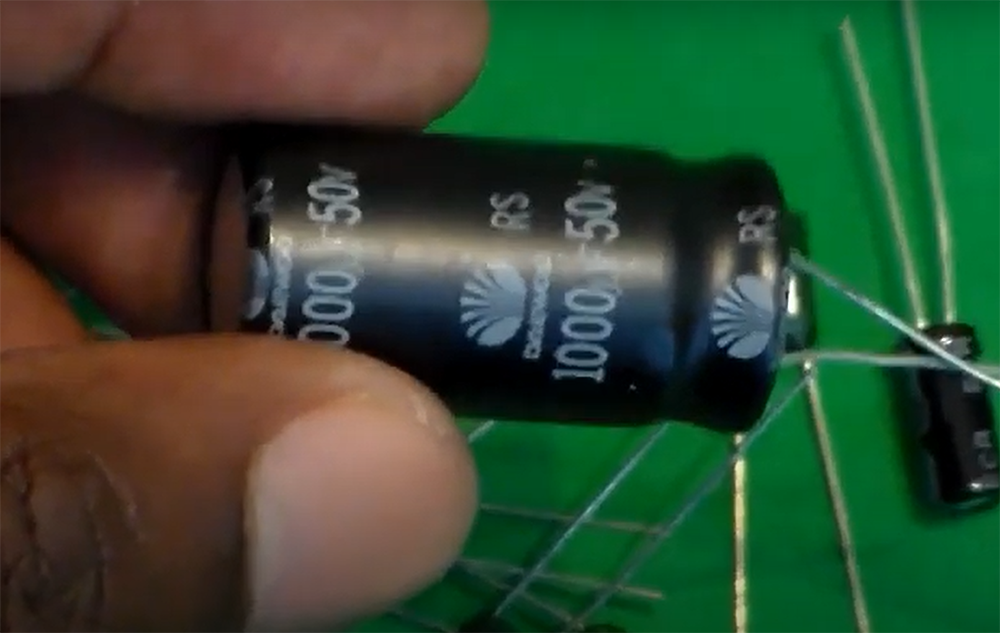Don’t pay much attention to the capitalization of the letters; it is common to see capacitors labeled with both “µF” and “UF”. , 

### Check the voltage rating

The voltage rating of a capacitor is the maximum amount of voltage that can be applied to the terminals without damaging the component. As we mentioned, this is usually printed on the side of the capacitor, along with the capacitance and other information. For example, a capacitor might be labeled “100V” and “0.47uF”. This means that the capacitor has a capacitance of 0.47 microfarads (uF) and a voltage rating of 100 volts.

It’s important to check the voltage rating of a capacitor before using it in your circuit. Applying too much voltage to a capacitor can damage or destroy the component.

It’s also important to note that capacitors can exhibit what’s known as “voltage dependence.” This means that their capacitance can change slightly when the voltage across them changes. For example, a capacitor with a value of 0.47 µF and a voltage dependence of ±20% will have a capacitance that ranges from 0.376 µF to 0.568 µF at 100 volts, but only from 0.392 µF to 0.544 µF at 50 volts. , , 

### Look for a tolerance value

The tolerance of a capacitor is the amount by which the capacitance can vary from the stated value. For example, a capacitor with a value of 0.47 µF and a tolerance of 20% can have a capacitance that ranges from 0.376 µF to 0.568 µF. The lower the tolerance, the more accurate the capacitance value will be.

Tolerance is usually indicated by a code printed on the side of the capacitor. The most common codes are J (±20%), K (±30%), M (±50%), and Z (±100%). The tolerance is usually printed on the side of the capacitor, next to the capacitance.

It’s important to consider tolerance when choosing a capacitor, because it will affect how well the component performs in your circuit. If you need an exact value for your circuit to work properly, you should choose a capacitor with a small tolerance (±0.01%, for example). However, if you have some flexibility in the values you use, you can choose a capacitor with a larger tolerance (±20%, for example)., , 

### Consider the polarity of the leads

When reading a capacitor, it’s also important to pay attention to the polarity of the leads. Most capacitors are polarized, meaning that they can only be connected one way in a circuit. The positive lead is usually marked with a “+” sign, while the negative lead is usually marked with a “-” sign. Some capacitors, such as electrolytic capacitors, can be damaged if they are connected backwards.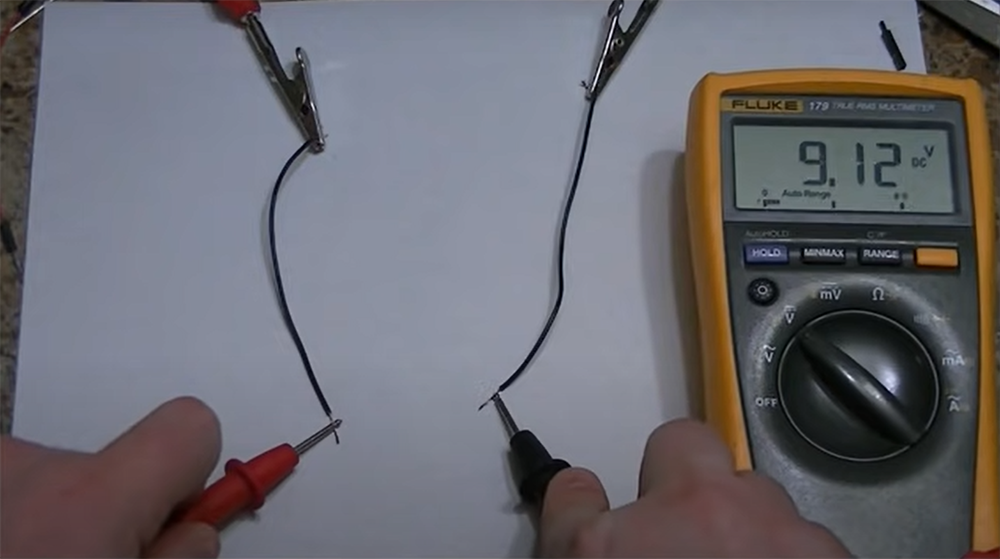Polarized capacitors must be connected in the correct direction in order to work properly. If you connect a polarized capacitor in the wrong direction, it will not function correctly (and could be damaged). Unpolarized capacitors can be connected in either direction and will still work properly. For example, if you’re using a capacitor to filter AC noise from a power supply, you’ll need to make sure that the positive lead of the capacitor is connected to the positive lead of the power supply and that the negative lead of the capacitor is connected to ground.  , , 

Now that you know the basics of reading a capacitor, let’s take a look at some common ways that capacitors are labeled.

### Write down the first two digits of the capacitance value

If the capacitor code consists of numbers and letters, the first two digits of the code will represent the capacitance value. Most capacitors will have a three-digit code printed on them. The first two digits are the value and the third digit is the multiplier.

If your capacitor shows only two numbers followed by a letter, that’s your capacitance value in picofarads (pF). The letter is your multiplier and you won’t need to perform any additional calculations.

### Use the third digit as a zero multiplier

If your code reads three numbers followed by a letter, the third number is your zero multiplier. This means that you’ll need to multiply the capacitance value by ten enough times to get the true value. For example, if your code reads 104K, you should add four 0 to the end of 104 to get 104,000.

However, there are some exceptions to that rule. If the third number is an 8, then you should multiply the number by 0.01. So, if your code reads 188K, you should multiply 18 by 0.01 to get the true value, which is 0.18.

If the third number is a 9, then you should multiply the number by 0.1. For example, if your code reads 188K, you should multiply 18 by 0.1 to get the true value, which is 0.18.

### Work out the capacitance units from the size

When you know the units a capacitor is measured in, this makes reading its value much easier. If you’re unsure about the units, look for context clues in the circuit diagram or other documentation. In some cases however, the size of the capacitor will give you a clue as to its capacitance units. For example, very small capacitors are usually measured in pico-farads (pF), while large capacitors tend to be in farads (F).

### How to read codes that contain letters

Not all codes start with numbers only, some will have letters too. These represent the tolerance of the capacitor, which is how accurate the capacitance value actually is. The most common letter you’ll see is ‘R’, which stands for ‘pF’. This means that the capacitance value of 6R2 will be around 6.2.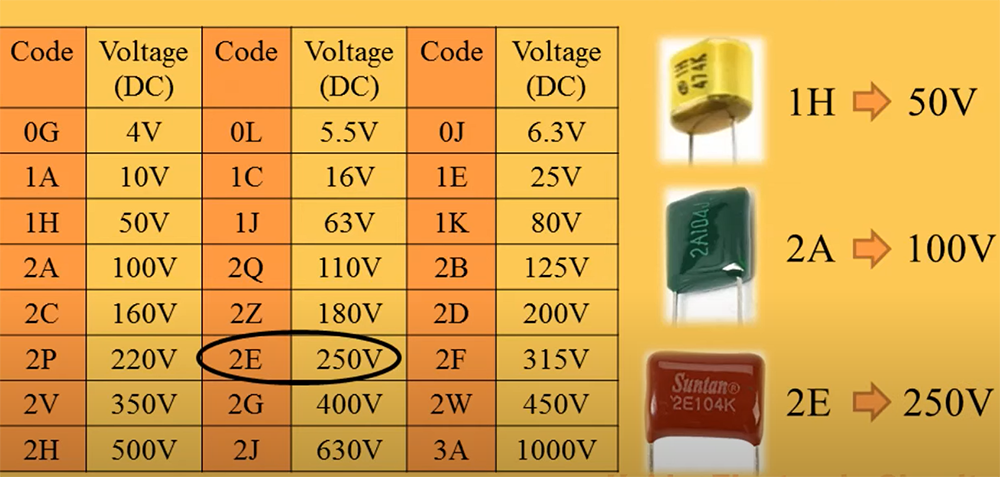Small letters like p, n, or u usually represent picofarads (pF), nanofarads (nF), or microfarads (uF) respectively. So, a code that reads n47 would be 0.47 nf.

Sometimes a code mixes a voltage value together with capacitance, for example 1A348. In this case, the voltage is given first (1A) and the capacitance is given second (348).

K or M are usually the highest multipliers you’ll see and they both represent thousand. So, a code that reads 104K would be 104,000 pF.

### Read the tolerance code on ceramic capacitors

Ceramic capacitors are one of the most common types of capacitor used in electronic circuits. They are made from a ceramic material, which has a high dielectric constant. This means that they can store a very large amount of electrical charge for their size. Ceramic capacitors are usually small and lightweight, making them ideal for use in portable electronic devices.

One disadvantage of ceramic capacitors is that they have a relatively low operating temperature range. This means that they can be damaged by exposure to excessive heat or cold. Another disadvantage is that they tend to be less reliable than other types of capacitor, such as electrolytic capacitors.

Being smaller, it’s no wonder the codes used to read their values are more compact. In fact, many times you’ll find a four-symbol code instead of the standard five digits. The first three digits in this code represent the capacitance, while the third symbol, a letter, represents the tolerance. For example, a ceramic capacitor with the code “223J” would have a value of 223 picofarads with a tolerance of +/- 5%.

As you can see, different letters represent different tolerance values. Let’s list them out along with their corresponding percentage:

• B: +/-0.1 pf
• C: +/-0.25 pf
• D: +/-0.5 pf
• F: +/-1%
• G: +/-2%
• J: +/-5%
• K: +/-10%
• M: +/-20%
• Z: +80%/-20%

As you can see, reading a ceramic capacitor is relatively easy once you know what to look for. Just remember that the first two digits represent the value and the third digit represents the tolerance. With this information in mind, you should be able to read any ceramic capacitor code without any trouble.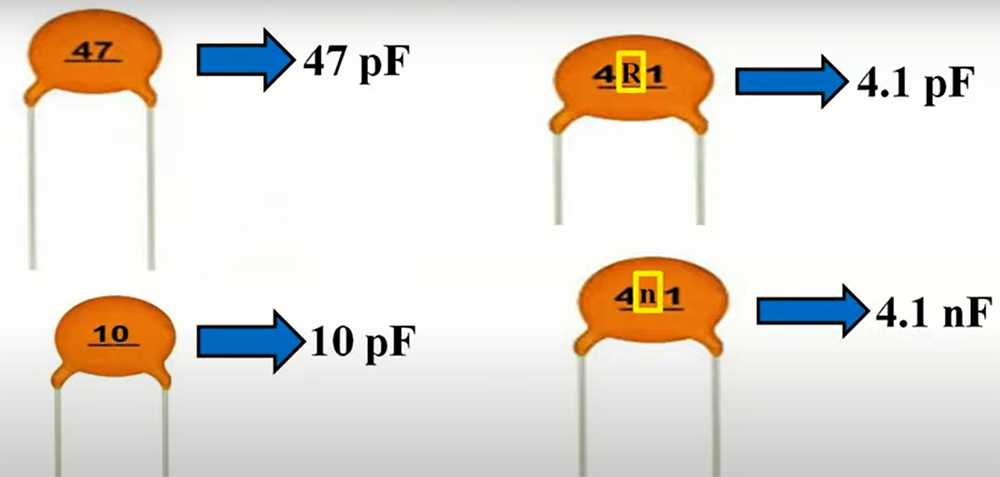Sometimes, tolerance is represented not only with a single letter but with a letter-number-letter combination. These are called “multiplier” codes and they’re used to indicate the tolerance of very large or small capacitors.

The first symbol in this code is always a letter and it represents the minimum temperature coefficient. For example, Z would be equal to 10ºC, Y to -30ºC and X to -55ºC.

Next is a number and it represents the maximum temperature. For example: 2 = 45ºC, 4 = 65ºC, 5 = 85ºC, 6 = 105ºC, 7 = 125ºC.

The last letter represents the variation in capacitance over the temperature range. Letter A is the most precise and it indicates a variation of +/-1%. Letter V is the least precise and it indicates a variation of +22/-82%.

### Interpret voltage codes

Finally, some capacitors also have a voltage code. To read it, you will need to consult a EIA chart. The voltage code will appear as a two digit code, consisting of a number and a letter. For example, if you see 2D, this means the capacitor is rated for use with up to 200 volts.

## FAQ

### How do I read a capacitor with a multimeter?

To read a capacitor with a multimeter, you’ll need to set the multimeter to the “capacitance” mode. Once you’ve done that, simply touch the leads of the capacitor to the corresponding terminals on the multimeter. The reading on the display will tell you the capacitance of the capacitor.

It’s important to note that most capacitors will have their value printed on them, so you can often just look for that instead of using a multimeter. However, if there’s no value printed or if you want to test an unknown capacitor, using a multimeter is definitely the way to go.

### What are the markings on a capacitor?

The markings on a capacitor are called “polarity markings”. They indicate the order of the layers inside the capacitor. The positive lead (longer leg) goes to the “+” side, and the negative lead (shorter leg) goes to the “-“.

If you’re still not sure which is which, there are a few other ways to tell. First, look for a stripe or dot on one of the leads. This indicates the negative lead. Second, look at the body of the capacitor. The longer lead will usually be closer to the ground (the big metal plate).

### What do the letters and numbers on a capacitor mean?

The letters and numbers on a capacitor indicate its value and tolerance. The value is measured in Farads (symbol: F) and the tolerance is indicated by a percentage sign. For example, if a capacitor has the markings “100nF 50V” it has a value of 100 nanofarads (0.0001 Farads) with a tolerance of plus or minus 50%.

To read a capacitor, you need to know two things: the value and the tolerance. The value is measured in Farads, while the tolerance is indicated by a percentage sign.

### How do you read capacitor voltage?

You can read capacitor voltage by using a multimeter. First, set the multimeter to the “DC Voltage” setting. Then, touch the black lead of the multimeter to the negative terminal of the capacitor, and touch the red lead of the multimeter to the positive terminal of the capacitor. The reading on the multimeter will tell you the voltage across the terminals of the capacitor.